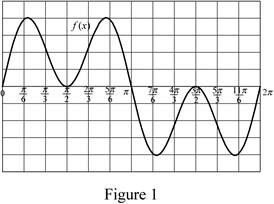# To Draw: the graph for the function f ( x ) = cos 2 x sin x . To Evaluate: The value of the integral function ∫ 0 2 π f ( x ) d x### Single Variable Calculus: Concepts...

4th Edition
James Stewart
Publisher: Cengage Learning
ISBN: 9781337687805### Single Variable Calculus: Concepts...

4th Edition
James Stewart
Publisher: Cengage Learning
ISBN: 9781337687805

#### Solutions

Chapter 5, Problem 38RE
To determine

## To Draw: the graph for the function f(x)=cos2xsinx.To Evaluate: The value of the integral function ∫02πf(x) dx

Expert Solution

The value of the integral function is 0.

### Explanation of Solution

Given information:

The function is f(x)=cos2xsinx.

The region lies between x=0 and x=2π.

Calculation:

Show the function as follows:

f(x)=cos2xsinx (1)

Substitute 0 for x in Equation (1).

f(0)=cos2(0)sin(0)=1×0=0

Substitute π6 for x in Equation (1).

f(π6)=cos2(π6)sin(π6)=0.75×0.5=0.38

Calculate the remaining values of the function as shown below.

Show the x and f(x) values as in Table (1).

 x cos2x sinx f(x) 0 1.00 0.00 0.00 π6 0.75 0.50 0.38 π3 0.25 0.87 0.22 π2 0.00 1.00 0.00 2π3 0.25 0.87 0.22 5π6 0.75 0.50 0.38 π 1.00 0.00 0.00 7π6 0.75 -0.50 -0.38 4π3 0.25 -0.87 -0.22 3π2 0.00 -1.00 0.00 5π3 0.25 -0.87 -0.22 11π6 0.75 -0.50 -0.38 2π 1.00 0.00 0.00

Table 1

Sketch the function as shown in Figure 1.Refer to Figure 1.

The value of the integral function 02πf(x)dx is 0.

Consider u=cosx (2)

Differentiate both sides of the Equation (2).

du=sinxdx (3)

Calculate the lower limit value of u using Equation (2).

Substitute 0 for x in Equation (2).

u=cos(0)=1

Calculate the upper limit value of u using Equation (2).

Substitute 2π for x in Equation (2).

u=cos(2π)=1

Substitute u for cosx and du for sinxdx in Equation (1) as shown below.

f(u)=u2(du)=u2du (4)

Find the integral value of the function as shown below.

02πf(x)dx=11(u2du)=(u33)11=(133133)=0

Therefore, the integral value of the function is 0.

### Have a homework question?

Subscribe to bartleby learn! Ask subject matter experts 30 homework questions each month. Plus, you’ll have access to millions of step-by-step textbook answers!